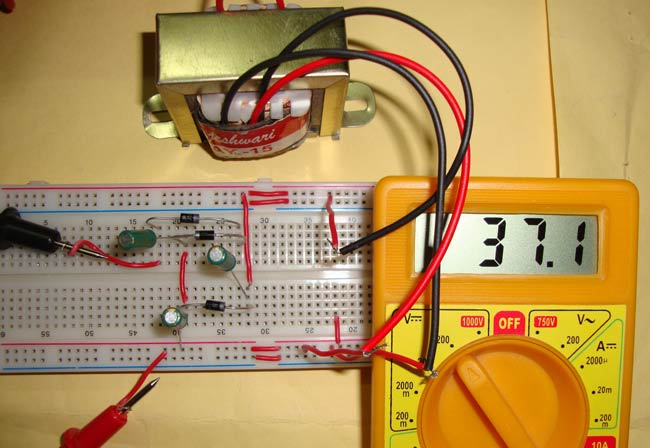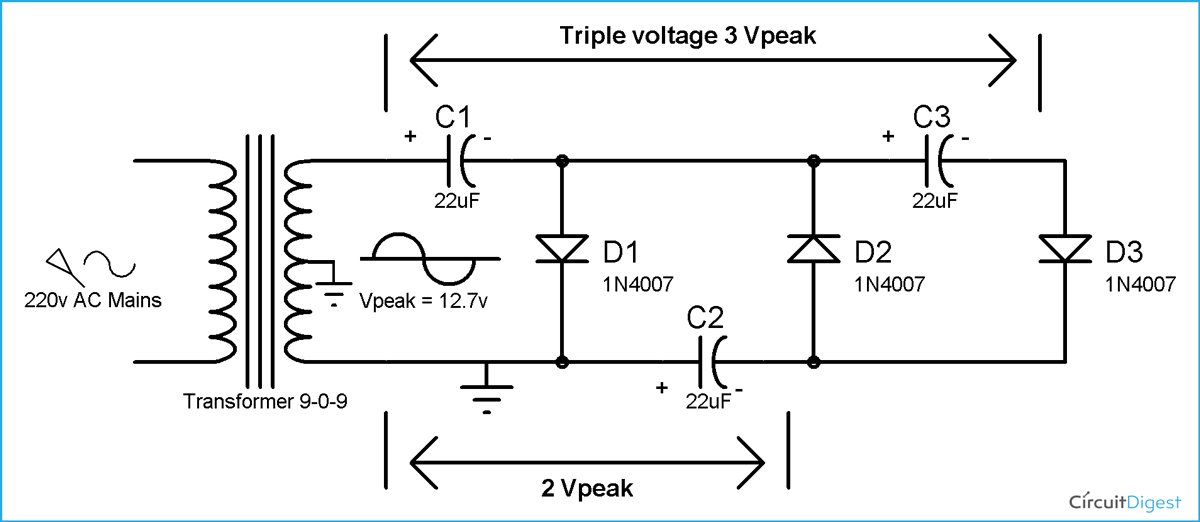# Voltage Tripler Circuit

Published  October 14, 2015   7
J
AuthorVoltage Tripler is the circuit where we get the thrice of the peak input voltage, like if the peak voltage of AC voltage is 5 volt, we will get 15 volt DC at the output. Generally transformers are there to step-up or step-down the voltage, but sometimes transformers are not feasible because of their size and cost. This kind of voltage tripler (voltage multiplier) can be built using few diodes and capacitors. These circuits are very useful where High DC voltage needs to be generated with Low AC voltage and low current is required, like in CRT (Cathode ray tubes) monitors in TV and computers. CRT monitor requires high DC voltage with low current.

### Components

• Diodes -3 (1N4007)
• Capaciotors- 22uf (3)
• Transformer (9-0-9)

### Voltage Tripler Circuit Diagram & ExplanationWe can extend the previous voltage doubler circuit, to create the voltage tripler circuit. In previous circuit we have used 555 timer to generate the square wave through DC, but in this circuit we have used AC (Alternate current) and just added one more diode and capacitor to triple the voltage.

We have used 9-0-9 transformers to step down the AC mains voltage (220v), so that we can demonstrated it on breadboard.

During the first positive half cycle of AC, Diode D1 get forward biased and capacitor C1 get charged through the D1. Capacitor C1 get charged up to the peak voltage of AC i.e. Vpeak.

During the negative half cycle of the AC, Diode D2 conducts and D1 reverse biased. D1 blocks the discharging of capacitor C1. Now the capacitor C2 charge with the combined voltage of capacitor C1 (Vpeak) and the negative peak of the AC voltage that is also Vpeak. So the capacitor C2 charge up to 2Vpeak volt.

During the second positive half cycle, Diode D1 and D3 conducts and D2 get reverse biased. In this way capacitor C2 charges the capacitor C3 up to the same voltage as itself, which is 2 Vpeak.

Now the capacitor C1 and C3 are in series and voltage across C1 is Vpeak and voltage across C3 is 2 Vpeak, so the voltage across the series connection of C1 and C3 is Vpeak+2Vpeak = 3 Vpeak, that how we get the Triple voltage of the peak value of AC. Although the voltage is not exactly thrice of the Peak voltage, because some voltage drops across the Diodes, so the resulting voltage would be:

Vout = 3*Vpeak – voltages drop across diodes

In our case we have used 9v as input voltage and got approx. 37.1v output voltage. 9v is the RMS value so the Vpeak value is 9*root 2 = 9*1.414 = 12.7 v.

So our output voltage should be:  12.7*3 = 38.1v

But we got approx. 37.1v, so approx. 38.1 – 37.1 = 1v has been dropped across diodes.

Disadvantage of this voltage tripler circuit is that Ripple frequency is very high and it very difficult to smooth the output, using the large value of capacitors can help reduce rippling. And the advantage is that we can generate very high voltage from a Low voltage power source.

Notes:

• Voltage won’t triple instantaneously but it will increase slowly and after some time, it will set to the Thrice of input voltage.
• The voltage rating of capacitor C2 and C3 should be at least twice the input voltage.
• The output voltage is not exactly the thrice of input voltage, it will be less than Input voltage. Like we got 37.1v for 12.7 Vpeak value of AC supply (9v is rms value, means Vpeak is 9*1.414 = 12.7v) input supply.

We can also generate much higher voltage and can get quadruple, 5 times, 6 times, 7 times and more, the voltage of the Peak AC voltage, by adding more diodes and capacitors.

Video

Submitted by Marcelo on Thu, 10/15/2015 - 21:45

### Corrente final do circuito

Qual a corrente de saída do circuito?

Submitted by Mike Higgins on Fri, 03/25/2016 - 01:54

### Oops!

The circuit as shown has one slight problem. You are shorting out one half of the secondary winding since the center tap and the lower end of the secondary winding both are shown as connected to ground.

Submitted by sagar on Tue, 11/08/2016 - 19:10

### can i use voltage tripler

can i use voltage tripler circuit with dc input?

Submitted by Mustapha on Sun, 03/05/2017 - 01:04